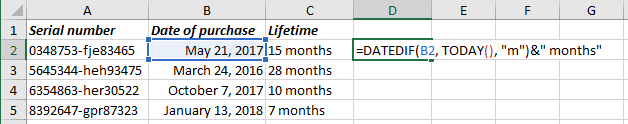# How to calculate a lifetime in Excel

Excel
Sometimes it is necessary to calculate a lifetime for some devices, things. Usually, a lifetime calculates in full months, for example, to check a warranty or guaranty period. Excel proposes a very useful formula that can help calculate that time.

The formula DATEDIF calculates days, months or years between two dates:

= DATEDIF (<start date>, <end date>, unit)

The parameter unit can be:

• "M" - The number of complete (full) months in the period.

For example, to calculate a lifetime:

= DATEDIF (<start date>, TODAY (), “m”)See also this tip in French: Comment calculer une durée de la vie dans Excel.